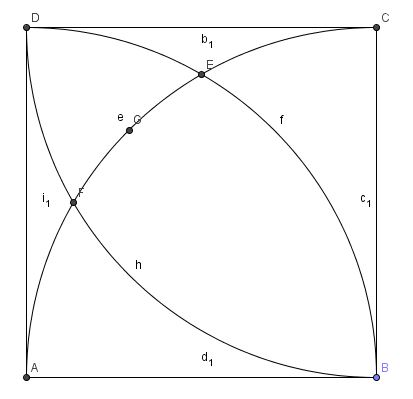# Bash or No Bash?

Geometry Level 4Square $ABCD$ has 3 quarter circles drawn on as shown above.

Points $E$ and $F$ are the intersections of the arcs. Point $G$ is the midpoint of arc $EF$. Find the value of $\angle EGF$ in degrees.

×